# Quiz 13: Overhead and Marketing Variances

Business

There is no answer for this question

Standard cost Standard cost is the cost the company expects would be incurred for direct materials, direct labor and for overhead. The standard cost may be determined by the company based on historical trends or based on current market situations. Company would determine the standard unit of raw material required to produce a product or the standard direct labor hours required to produce a single product. Also in case of direct material the material cost per unit and in case of labor cost direct labor cost per hour is determined in the standard costs. Labor variance When the actual labor cost differs from that of standard cost the difference in cost is termed as labor variance. The labor variance could arise due to different price for one hour of direct labor or due to different labor hour actually used to produce a product which is known as efficiency variance. Formula to calculate labor rate variance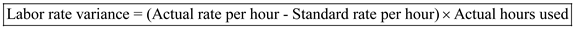Formula to calculate labor efficiency variance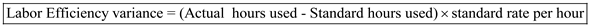Variable overhead variance When the actual variable overhead differs from that of standard cost the difference in cost is termed as variable overhead variance. Variable overhead variance could arise due to different price for one hour of direct labor or due to different labor hour actually used to produce a product which is known as efficiency variance. Formula to calculate overhead spending variance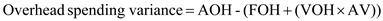Formula to calculate overhead efficiency variance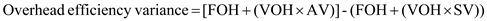Fixed overhead variance Fixed overhead budget variance is when there is difference between actual fixed overhead and budgeted fixed overhead. When actual overhead incurred is lower than budgeted overhead the variance is favorable and if actual overhead incurred is higher than budgeted overhead the variance is unfavorable. Formula to calculate overhead volume variance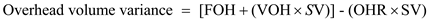Using the overhead volume variance we can determine the standard volume and thus calculate the excess capacity.Thus, standard volume is 640,000 Calculate excess capacity as shown belowThus, company had excess capacity of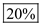There is no answer for this question